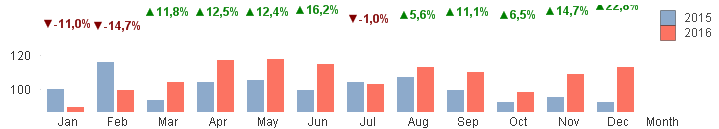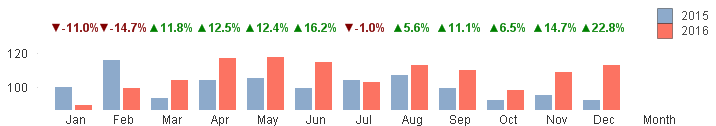QlikView Documents

Documents for QlikView related information.

Custom Data Points To Create Values Above Bars In Bar ChartEmployee

Custom Data Points To Create Values Above Bars In Bar Chart

Recently I can across a case where a customer wanted to display the difference in sales per month, when comparing two years. The idea was to use a Combo Chart, utilizing bars for the sales numbers and dots for the percentage changes. The problem naturally was that the dots are rendered based on their relative value, meaning that the percentages are shown in a some what skewed line.To resolve this I generated customized data points through the Dual() function, so that the presentation value still looks accurate but with underlying numbers that render the values on the same line.The original calculation is left in a expression without any visual presentation. In the my example this is called "%Diff". The only aggregation change I made to this was to add the required rounding to two decimals. Being a percentage value, it required multiplying with 100 before the rounding.

Round((<Original Expression>*100, 0.1)

The original chart uses the chart properties number format pattern "▲# ##0,0%;▼-# ##0,0%" to accomplish the up and down arrows. For the custom data point value, the format variable will not be an option, so the formatting has to be done in an other way. In the new (and additional) expression called "Change" the formatting is managed through a simple If statement that refer to the %Diff expression. This will be the the text part of the customized Dual value.

If([%Diff]>0, '▲'&[%Diff]&'%', '▼'&[%Diff]&'%')

Then numerical value in the customized data point should be unique on each data point. A simple way to get a new value for each data point is to use RowNo() function. The only problem with this is that the result is a integer, with naturally puts all values on different lines in chart context. By adding the row number to a large integer value (10^9) and dividing the value with a larger value (10^10) the result is unique number for each row, which touch on the 0.1 line.

(1000000000+RowNo(TOTAL))/10000000000

With the Dual() function the two custom component can now be combined into a custom value, which generates a straight line for the dot data value points.

Dual( If([%Diff]>0, '▲'&[%Diff]&'%', '▼'&[%Diff]&'%'),

(1000000000+RowNo(TOTAL))/10000000000

)

Note, in the sample chart is not very high. To push the text labels into visible area I have set the Static Max to 0.8.

Labels (3)

• Layout & Visualizations

Version history
Revision #:
1 of 1
Last update:
‎2016-09-08 04:24 AM
Updated by: